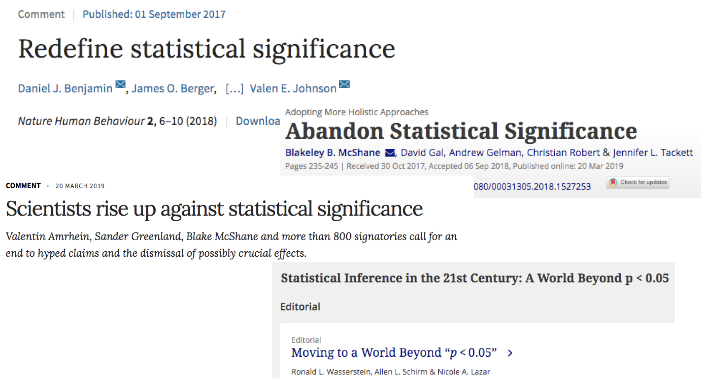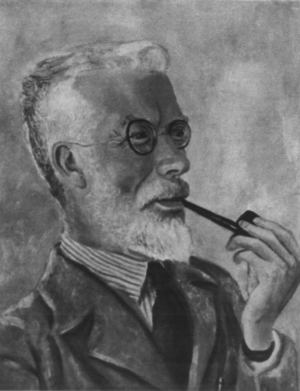30th June, 2019

## Why should we care about statistics?

• Uncertainty is integral to both science and life
• Statistics is the discipline that deals with quantifying uncertainty
• In contrast to how it is usually taught, statistics is a messy discipline full of opposing viewpoints

• In the 20th century and beyond, there were 3 big traditions in statistics
• They continue to influence our thinking profoundly

## Historical context## Significance? What significance?

• Before 1940, significance testing was virtually non-existent
• By 1955, 80% of empirical articles reported significance tests
• Today, the figure is close to 100% (see Gigerenzer, 1993)

“[Statisticians] have already overrun every branch of science with a rapidity of conquest rivalled only by Atilla, Mohammed, and the Colorado beetle.”

• Kendall (1942, p.69)

## Ronald Fisher• Father of modern statistical inference
• Randomization, design of experiments
• $$p$$-value, $$\alpha$$ level, null hypothesis, analysis of variance
• Maximum likelihood estimation (Stigler, 2007)
• The “greatest of Darwin’s successors” (Edwards, 2011)

## Neyman-Pearson

• Jerzy Neyman and Egon Pearson put Fisher’s ideas on a rigorous mathematical basis
• Fisher didn’t like it, and a personal feud began that lasted until his death

• Some concepts Neyman & Pearson introduced were
• The alternative hypothesis
• Type I ($$\alpha$$) and Type II ($$\beta$$) errors
• Statistical power
• Confidence intervals
• Decision theoretic foundation of hypothesis testing